# Todays lecture Powers of ten Scientific method Powers

• Slides: 12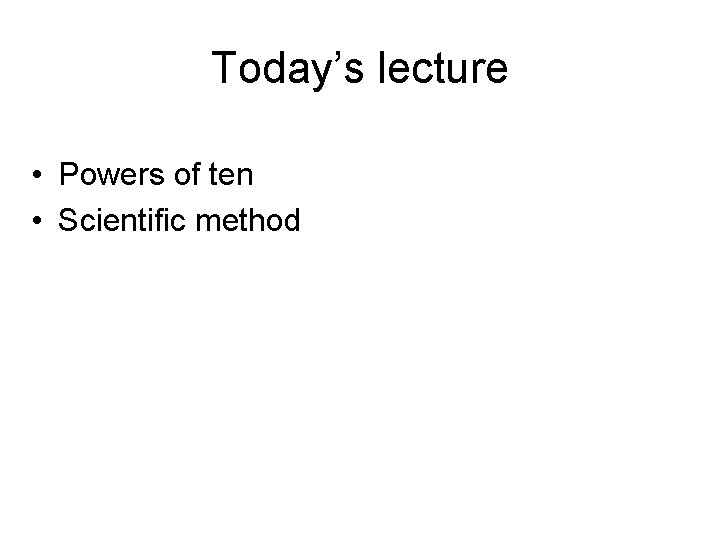Today’s lecture • Powers of ten • Scientific methodPowers of ten are shorthand for writing very large numbers 100 = 1 One 101 = 10 Ten (deca) 102 = 100 Hundred (centa) 103 = 1, 000 Thousand (kilo) 106 = 1, 000 Million (mega) 109 = 1, 000, 000 Billion (giga) 1012 = 1, 000, 000 Trillion (tera) 1015 = … Quadrillion (peta) 1054 = … Septendecillion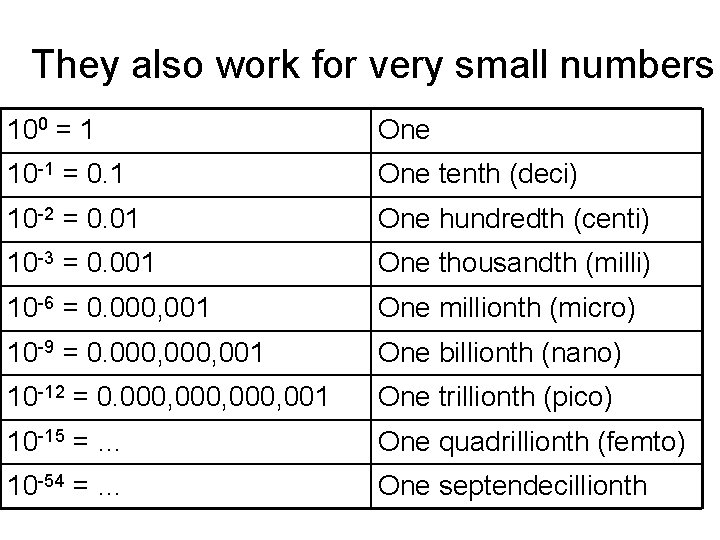They also work for very small numbers 100 = 1 One 10 -1 = 0. 1 One tenth (deci) 10 -2 = 0. 01 One hundredth (centi) 10 -3 = 0. 001 One thousandth (milli) 10 -6 = 0. 000, 001 One millionth (micro) 10 -9 = 0. 000, 001 One billionth (nano) 10 -12 = 0. 000, 001 One trillionth (pico) 10 -15 = … One quadrillionth (femto) 10 -54 = … One septendecillionth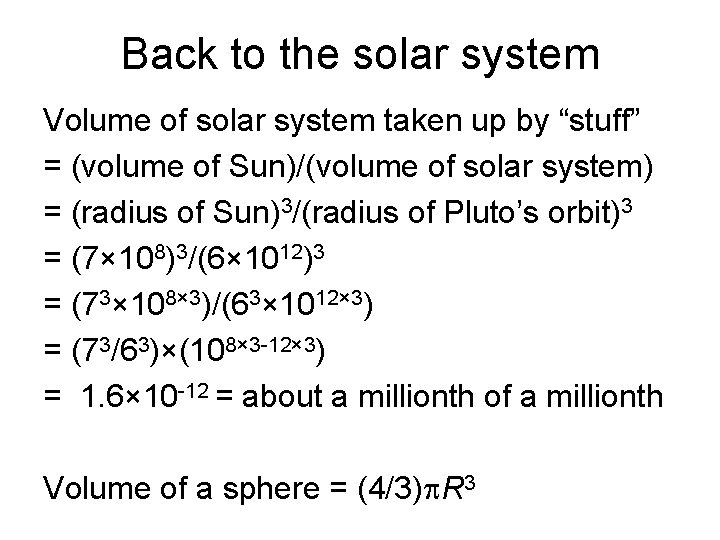Back to the solar system Volume of solar system taken up by “stuff” = (volume of Sun)/(volume of solar system) = (radius of Sun)3/(radius of Pluto’s orbit)3 = (7× 108)3/(6× 1012)3 = (73× 108× 3)/(63× 1012× 3) = (73/63)×(108× 3 -12× 3) = 1. 6× 10 -12 = about a millionth of a millionth Volume of a sphere = (4/3)p. R 3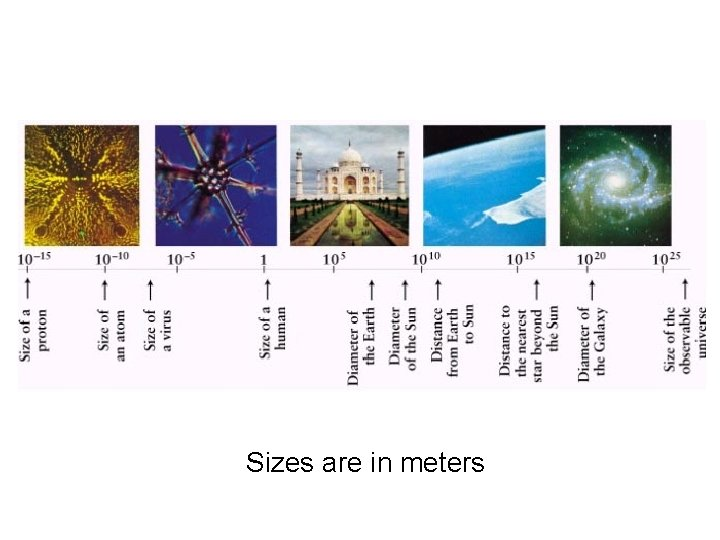Sizes are in metersWatch Movie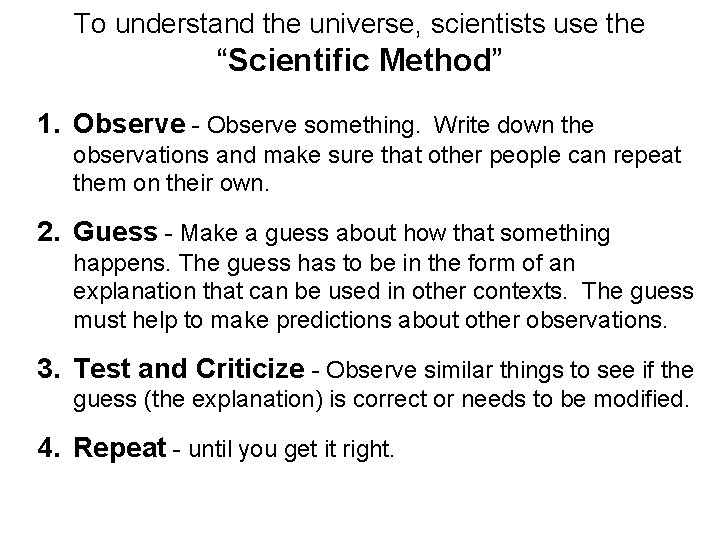To understand the universe, scientists use the “Scientific Method” 1. Observe - Observe something. Write down the observations and make sure that other people can repeat them on their own. 2. Guess - Make a guess about how that something happens. The guess has to be in the form of an explanation that can be used in other contexts. The guess must help to make predictions about other observations. 3. Test and Criticize - Observe similar things to see if the guess (the explanation) is correct or needs to be modified. 4. Repeat - until you get it right.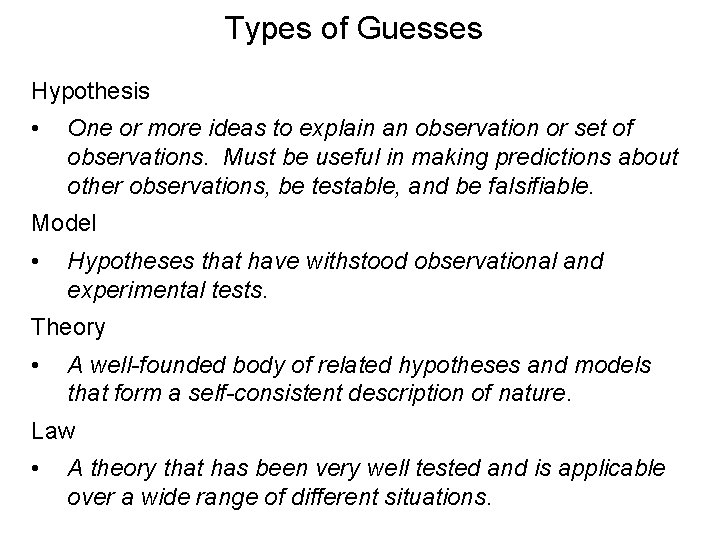Types of Guesses Hypothesis • One or more ideas to explain an observation or set of observations. Must be useful in making predictions about other observations, be testable, and be falsifiable. Model • Hypotheses that have withstood observational and experimental tests. Theory • A well-founded body of related hypotheses and models that form a self-consistent description of nature. Law • A theory that has been very well tested and is applicable over a wide range of different situations.Which are valid scientific hypotheses? 1. Any two objects dropped from the same height above the surface of the moon will hit the lunar surface at the same time. 2. All horses are brown. 3. Our universe is surrounded by another, larger universe, with which we can have absolutely no contact.Science, religion, and pseudo-science • Scientific theories are experimentally verifiable (or falsifiable) and predictive. They address how questions (e. g. How do stars form? When will the next lunar eclipse be? ) • Religious and ethical statements are (generally) not verifiable. They address why questions (e. g. Why does the Universe exist? What kind of life is worth living? ). These are not intrinsically less worthwhile than scientific inquiries, they are simply addressing different questions. • Pseudo-science theories pretend to be scientific but are either not falsifiable (e. g. séances, ) or supporters or use anecdotal evidence to support claims (e. g. astrology, ‘creation’ science).Skepticism and Truth • Role of skepticism. An essential part of the scientific method. Scientists always question the basis for an scientific assertion. This is often considered ‘impolite’ behavior in social settings, but is not impolite in scientific discussion. • ‘What is truth? ’ - Scientific theories are not statements of truth. They are the best available explanation for observed facts, but are subject to revision or falsification. Example: Gravitation (Newton vs. Einstein). • Scientists must be able to admit that they are wrong.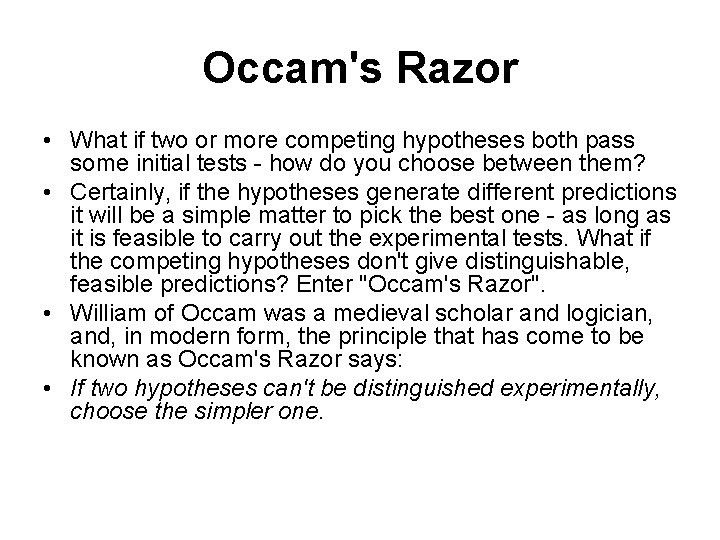Occam's Razor • What if two or more competing hypotheses both pass some initial tests - how do you choose between them? • Certainly, if the hypotheses generate different predictions it will be a simple matter to pick the best one - as long as it is feasible to carry out the experimental tests. What if the competing hypotheses don't give distinguishable, feasible predictions? Enter "Occam's Razor". • William of Occam was a medieval scholar and logician, and, in modern form, the principle that has come to be known as Occam's Razor says: • If two hypotheses can't be distinguished experimentally, choose the simpler one.